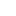• ### DEED: A general quantization scheme for saving bits in communication

分类： 数学 >> 控制和优化 提交时间： 2020-06-16Tian Ye Peijun Xiao Ruoyu Sun

摘要：Quantization is a popular technique to reduce communication in distributed optimization. Motivated by the classical work on inexact gradient descent (GD) \cite{bertsekas2000gradient}, we provide a general convergence analysis framework for inexact GD that is tailored for quantization schemes. We also propose a quantization scheme Double Encoding and Error Diminishing (DEED). DEED can achieve small communication complexity in three settings: frequent-communication large-memory, frequent-communication small-memory, and infrequent-communication (e.g. federated learning). More specifically, in the frequent-communication large-memory setting, DEED can be easily combined with Nesterov's method, so that the total number of bits required is $\tilde{O}( \sqrt{\kappa} \log 1/\epsilon )$, where $\tilde{O}$ hides numerical constant and $\log \kappa$ factors. In the frequent-communication small-memory setting, DEED combined with SGD only requires $\tilde{O}( \kappa \log 1/\epsilon)$ number of bits in the interpolation regime. In the infrequent communication setting, DEED combined with Federated averaging requires a smaller total number of bits than Federated Averaging. All these algorithms converge at the same rate as their non-quantized versions, while using a smaller number of bits.

同行评议状态:待评议

• ### On The Birch and Swinnerton-Dyer Conjecture for CM Elliptic Curves over Q

分类： 数学 >> 代数与数论 提交时间： 2016-05-19LI, YONGXIONG LIU, YU Tian, Ye

摘要：For CM elliptic curve over rational field with analytic rank one, for any potential good ordinary prime p, not dividing the number of roots of unity in the complex multiplication field, we show the p-part of its Shafarevich-Tate group has order predicted by the Birch and Swinnerton-Dyer conjecture.

同行评议状态:待评议